Courses

# Fluid Dynamics And Flow Through Pipes - MCQ Test 2

## 30 Questions MCQ Test RRB JE for Mechanical Engineering | Fluid Dynamics And Flow Through Pipes - MCQ Test 2

Description
This mock test of Fluid Dynamics And Flow Through Pipes - MCQ Test 2 for Mechanical Engineering helps you for every Mechanical Engineering entrance exam. This contains 30 Multiple Choice Questions for Mechanical Engineering Fluid Dynamics And Flow Through Pipes - MCQ Test 2 (mcq) to study with solutions a complete question bank. The solved questions answers in this Fluid Dynamics And Flow Through Pipes - MCQ Test 2 quiz give you a good mix of easy questions and tough questions. Mechanical Engineering students definitely take this Fluid Dynamics And Flow Through Pipes - MCQ Test 2 exercise for a better result in the exam. You can find other Fluid Dynamics And Flow Through Pipes - MCQ Test 2 extra questions, long questions & short questions for Mechanical Engineering on EduRev as well by searching above.
QUESTION: 1

Solution:

Ans. (b)

QUESTION: 2

Solution:

Ans. (b)

QUESTION: 3

### Assertion (A): Two table tennis balls hang parallelly maintaining asmall gap between them. If air is blown into the gap between the balls,the balls will move apart.  Reason (R): Bernoulli's theorem is applicable in this case.

Solution:

Ans. (c)

QUESTION: 4

Assertion (A): Bernoulli's equation is an energy equation.
Reason (R): Starting from Euler's equation, one can arrive at Bernoulli's equation.

Solution:

Ans. (b) Starting from Euler's equation, one can arrive at Bernoulli's equation. And
we know that Euler equation is a momentum equation and integrating Euler
equation we can arrive at Bernoulli’s equation.

QUESTION: 5

Consider the following assumptions:
1. The fluid is compressible
2. The fluid is inviscid.
3. The fluid is incompressible and homogeneous
4. The fluid is viscous and compressible.
The Euler's equation of motion requires assumptions indicated in :

Solution:

Ans. (b)

QUESTION: 6

How is the velocity coefficient Cv, the discharge coefficient Cd, and thecontraction coefficient Cc of an orifice related?

Solution:

Ans. (c)

QUESTION: 7

Match List-I (Measuring Devices) with List-II (Measured Parameter) and select the correct answer using the codes given below: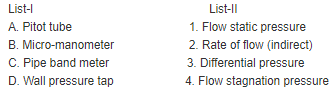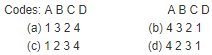Solution:

Ans. (b)

QUESTION: 8

Match List-I with List-II and select the correct answer using the codes given below the lists: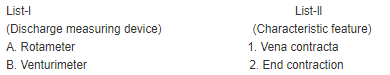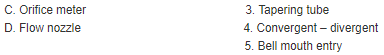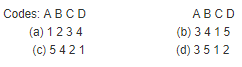Solution:

Ans. (b)

QUESTION: 9

Which one of the following conditions will linearize the Navier-Stokes equations to make it amenable for analytical solutions?

Solution:

Ans. (c)

QUESTION: 10

Assertion (A) : A cylinder, partly filled with a liquid is rotated about itsvertical axis. The rise of liquid level at the ends is equal to the fall ofliquid level at the axis.
Reason (R) : Rotation creates forced vortex motion.

Solution:

Ans. (b)

QUESTION: 11

Assertion (A): Head loss for sudden expansion is more than the head loss for a sudden contraction for the same diameter ratio.
Reason (R): Head loss varies as the square of the upstream and downstream velocities in the pipe fitted with sudden expansion or sudden contraction.

Solution:

Ans. (c)

QUESTION: 12

Which one of the following statements relates to expression ' ρ vc'?

Solution:

Ans. (d)

QUESTION: 13

Velocity of pressure waves due to pressure disturbances imposed in aliquid is equal to:

Solution:

Ans. (a)

QUESTION: 14

For maximum transmission of power through a pipe line with totalhead H, the head lost due to friction hf is given by:

Solution:

Ans. (b)

QUESTION: 15

Point A of head 'HA' is at a higher elevation than point B of head 'HB'.The head loss between these points is HL. The flow will take place.

Solution:

Ans. (c) Flow may take place from lower elevation to higher elevation. Everyday
we are pumping water to our water tank.
If flow is from point 1 to point 2 then
Total head at point 1 = Total head at point 2 + loss of head between 1 and 2
If flow is from point A to point B then
Total head at point A (HA) = Total head at point B (HB) + Loss of head between
A and B (HL)
If flow is from point B to point A then
Total head at point B (HB) = Total head at point A (HA) + Loss of head between
B and A (HL)

QUESTION: 16

If energy grade and hydraulic grade lines are drawn for flow through an inclined pipeline the following 4 quantities can be directly observed:
Starting from the arbitrary datum line, the above types of heads will:

Solution:

Ans. (d) Starting from the arbitrary datum line, the heads in sequence be in the sequence will be 3-datum head, 1-static head 4-velocity head, and 2- friction head.

QUESTION: 17

If coefficient of contraction at the vena contract is equal to 0.62, thenwhat will be the dynamic loss coefficient in sudden contraction in airconditioningduct?

Solution:

Ans. (b)

QUESTION: 18

Which one of the following statements is correct?

Solution:

Ans. (c)

QUESTION: 19

For a circular channel, the wetted parameter (where R = radius of circular channel, θ = half the angle subtended by the water surface atthe centre) is given by:

Solution:

Ans. (c)

QUESTION: 20

The pressure drop in a pipe flow is directly proportional to the meanvelocity. It can be deduced that the

Solution:

Ans. (a)

QUESTION: 21

How does the head loss in turbulent flow in pipe vary?

Solution:

Ans. (c) Head loss in the turbulent flow is Approximately proportional to square of
velocity. But Head loss in the Laminar flow is as proportional to velocity.

QUESTION: 22

Aging of pipe implies

Solution:

Ans. (d)

QUESTION: 23

The frictional head loss in a turbulent flow through a pipe varies

Solution:

Ans. (b) Frictional head loss in turbulent flow varies directly as the square of average velocity.

QUESTION: 24

Assertion (A): Nature of the fluid flow in a pipe does not dependentirely on average velocity but it actually a function of the Reynoldsnumber.
Reason (R): Reynolds number depends not only on average velocity butalso on the diameter of the pipe and kinematic viscosity of the fluid.

Solution:

Ans. (a) Reynold’s number decides the fluid flow is laminar or turbulent, i.e  Nature of fluid flow Re =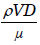QUESTION: 25

Which one of the following statements is true of fully developed flowthrough pipes?

Solution:

Ans. (a) For fully developed flow through pipes, the flow is parallel, has no inertia effect. The pressure gradient is of constant value and the pressure force is balanced by the viscous force.

QUESTION: 26

A right circular cylinder is filled with a liquid upto its top level. It isrotated about its vertical axis at such a speed that halt the liquid spillsout then the pressure at the point of intersection of the axis and bottom surface is:

Solution:

Ans. (d)

QUESTION: 27

Both free vortex and forced vortex can be expressed mathematically as functions of tangential velocity V at the corresponding radius r. Freevortex and forced vortex are definable through V and r as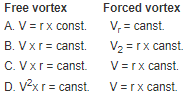Solution:

Ans. C. Free vortex can be expressed mathematically as Vx r = constant and the
forced votex as V = r x constant.

QUESTION: 28

The Bernoulli’s equation refers to conservation of

Solution:
QUESTION: 29

If the Reynolds number is less than 2000, the flow in a pipe is

Solution:
QUESTION: 30

If a fluid jet discharging from a 50 mm diameter orifice has a 40 mm diameter at its vena contracta, then its coefficient of contraction willbe:

Solution:

Ans. (b)